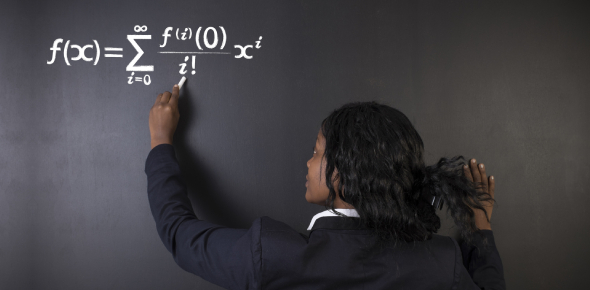20 Questions | Attempts: 1704
ShareSettingsHow good you're at solving math word problems? Check out these quiz questions with answers created for grade 6th students and evaluate your understanding of Math. Here, you'll be asked questions related to subtraction, addition, LCM, HCF, and factors. If you think this quiz is something you can give your precious time to, then go for it. Students up to class 8th can also play this quiz and make their Math basics stronger.

• 1.
A stationary store had 5,300 greeting cards. If they sold 3,710 cards, how many cards are left?
• A.

2,410 cards

• B.

1,690 cards

• C.

1,590 cards

• D.

1,591 cards

• 2.
What is the greatest common factor of 48 and 72?
• A.

9

• B.

24

• C.

12

• D.

144

• 3.
Which of these is NOT a factor pair of 225?
• A.

( 15 , 15 )

• B.

( 1 , 225 )

• C.

( 15, 4 )

• D.

( 75, 3 )

• 4.
• A.

Sum

• B.

Difference

• C.

Product

• D.

Option 4

• 5.
What do you call the answer in subtraction?
• A.

Sum

• B.

Difference

• C.

Product

• D.

Quotient

• 6.
Which of these is NOT a keyword in solving Addition word problems?
• A.

Fewer

• B.

Increase

• C.

Combined

• D.

Sum

• 7.
Which of these is a keyword in solving Subtraction word problems?
• A.

Increase

• B.

how many more

• C.

Total

• D.

Combined

• 8.
Which of these numbers is a factor of 78?
• A.

9

• B.

12

• C.

13

• D.

15

• 9.
Which of these numbers is NOT a factor of 99?
• A.

1

• B.

9

• C.

11

• D.

15

• 10.
Which of these numbers is a common multiple of 12 and 30?
• A.

30

• B.

40

• C.

50

• D.

60

• 11.
Which of these numbers is a common multiple of 8 and 12?
• A.

36

• B.

60

• C.

72

• D.

84

• 12.
What is the greatest common factor of 36 and 45?
• A.

5

• B.

9

• C.

15

• D.

225

• 13.
Mrs. Smith had 12 donuts. She bought 25 more. How many does she have now?
• A.

37 donuts

• B.

13 donuts

• C.

63 donuts

• D.

47 donuts

• 14.
What is the Least Common Multiple of 3 and 9?
• A.

3

• B.

6

• C.

9

• D.

18

• 15.
What is the Least Common Multiple of 8 and 10?
• A.

2

• B.

10

• C.

80

• D.

40

• 16.
Jake makes \$10 dollars an hour and Judy makes \$15 dollars an hour. What is the smallest check they can receive so that both their earnings match?
• A.

40

• B.

45

• C.

30

• D.

40

• 17.
Which of these is a factor pair of 72?
• A.

( 2 , 37 )

• B.

​​​​​​​ ( 3 , 24 )

• C.

( 2 , 72 )

• D.

​​​​​​​( 4 , 19 )

• 18.
What is the sum of 84,291 and 7,095?
• A.

91,381

• B.

91,385

• C.

91,382

• D.

91,386

• 19.
During June and July, a total of 25,150 visitors went to the Grand Canyon National Park. If 48,924 visitors went to the park in June, how many visitors went in July?
• A.

23,778

• B.

23,890

• C.

23,775

• D.

23,776

• 20.
A total of 3,718 tickets were sold for a skating show. Of that total, 1,279 were adult tickets. The remaining tickets were child tickets. How many child tickets were sold?
• A.

2,439 tickets

• B.

2,561 tickets​​​​​​

• C.

3,439 tickets

• D.

4,997 tickets

## Related TopicsBack to top
×

Wait!
Here's an interesting quiz for you.